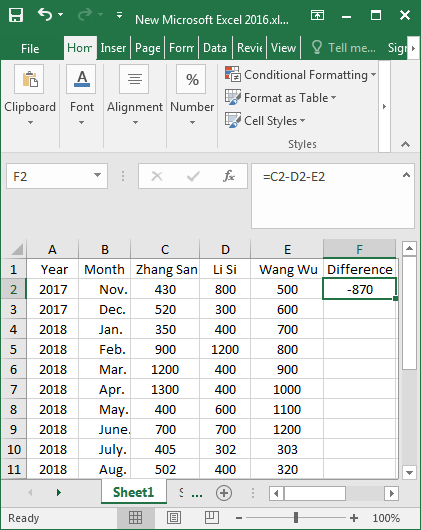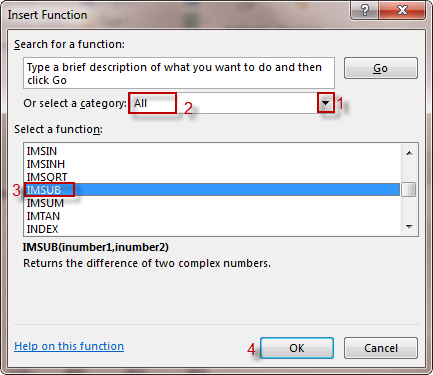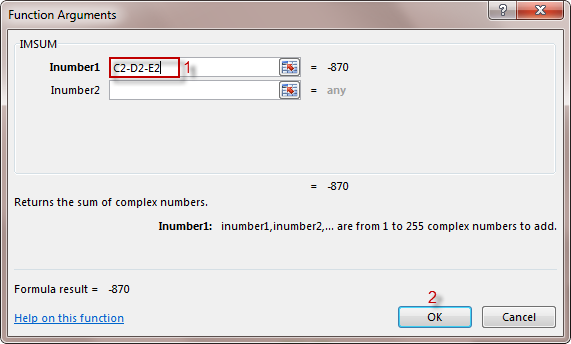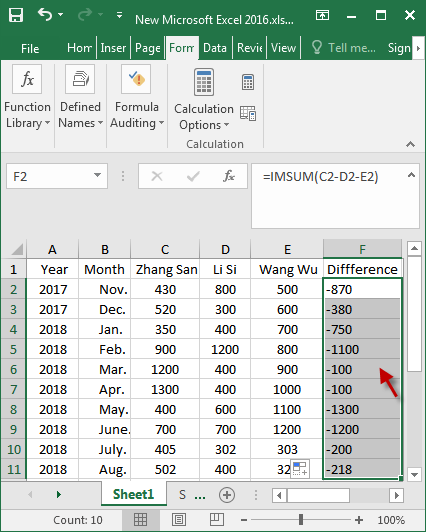• Blog>
• Office>
• 2 Ways to Calculate the Difference between the Data in Excel 2016

# 2 Ways to Calculate the Difference between the Data in Excel 2016Excel is a panacea, you can do anything with it. For some accountants, facing a big push data that is needed to figure it out. At this time, you can’t avoid the use of various operations, such as addition, subtraction, multiplication, division, average, and so on. This article will show you how to use excel to calculate the difference between the data in Excel 2016.

Even if you are a novice to learn excel, and you have to count the data in the table, but do not know what method can be used to quickly and easily calculate the difference of the data, that is ok, as long as you read this article, you can also calculate smoothly the data in Excel 2016.

## 2 ways to calculate the difference of the data in Excel 2016

### Way 1: Type the formula to calculate the difference

Step 1: Type the formula “=C2-D2-E2” in the F2 cell and press Enter.Step 2: At this time, the difference of C2:E2 appears in the F2 cell, and then move the mouse pointer to the lower right corner of the F2 cell. When the mouse turns into a black cross “+“, hold down the left button of the mouse and drag it down.Step 3: When you’re done, you can see that all the results will be displayed.### Way 2: Insert function to calculate the difference

Step 1: Select the F2 cell and execute the Formulas > Insert Function command.Step 2: When pops up the Insert Function dialog box, click the inverted triangle in the right of the select a category, select All in the drop-down menu. In the Select a function field, select IMSUB function and click OK.Step 3: When the Function Arguments dialog appears, type the C2-D2-E2 in Inumber1 and click OK.Step 4: At this point, the difference of F2 is solved, and then the cursor is placed at the lower right corner of the F2 cell, which turns into a cross “+“. Pressing the left button of the mouse, drag it down. After releasing the mouse, the difference of all data is completed.